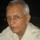Coffee Room
Discuss anything here - everything that you wish to discuss with fellow engineers.
12893 Members
Join this group to post and comment.# What is the difference between efficiency and gain?

can anyone explain the difference between efficiency and gain with detail?

please give me technical answer ..Input -> System -> Output

Output/Input x 100 = %Efficiency

1 kg diesel put into an engine say gives an output of 4.6 KWHr. ! kg diesel gives 10000 KCal energy. 4.6 KWHr is equal to 4000 KCals.

So, Efficiency = 100x(4000/10000)= 40%

Gain = Input-Output

There is 5 litres water at 50 deg C in an open vessel on a stove. It is losing 5 KCal/min by radiation and evaporation. If 10 KCal/min is given to the vessel by the stove, then       the net heat input into the water is 10-5=5KCal/min.

Gain = 10-5 =5 Kcal/min. The water will heat up at the rate of 5 deg C/minute.

These are rather quick and dirty examples.Take the water example 10 KCal/ Min was put in. What was the output? Not the 5 Kcal lost by radiation and evaporation but the % KCal gained by the water. So efficiency is 100x(5/10)=50%.

Unfortunately, the loss = gain so the % was the midpoint in this case. The important thing is to know what the actual output is.I apologise for an arithmetic error in my first post. 5 Kcal/min added to 5 litres of water will increase the temperature by 5/5=10C/min and not 50C.

Share this content on your social channels -